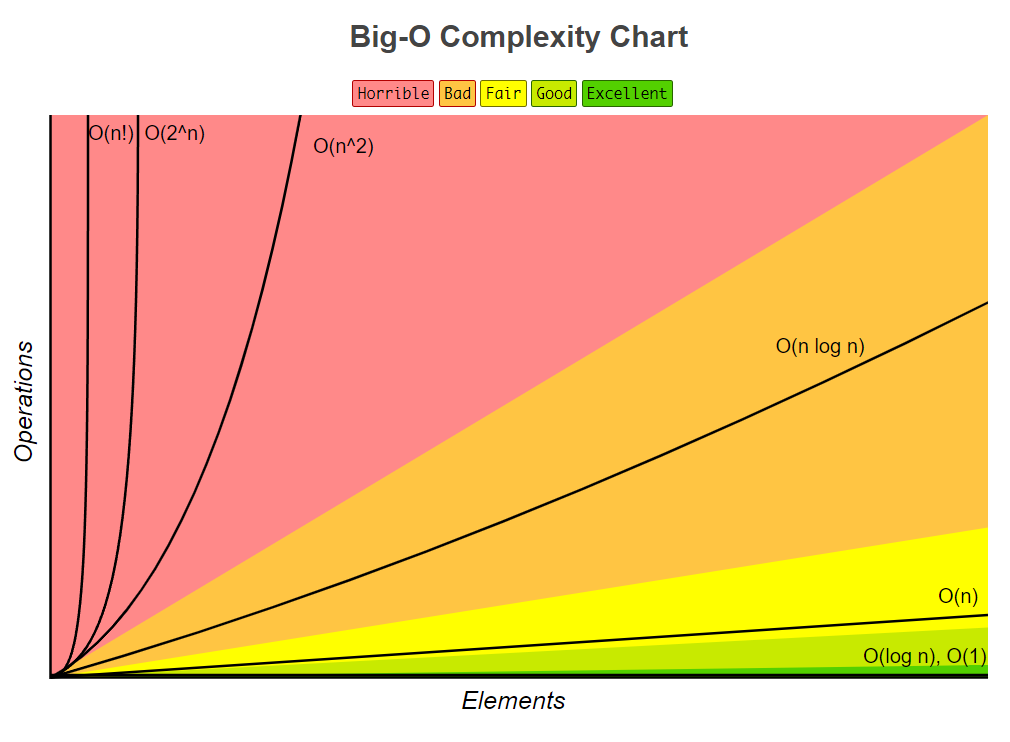# Big-O Notation §

Big O is useful to write code considering optimization.

Big- Upper Bound

### Math §

Definition from MATH119

Given two functions and , we say that “ is of order as ” and write if there exists a constant (greater than zero) such that on some interval around (although the point itself may be excluded from the interval, since the idea is to describe the behaviour of in the limit as we approach ).

In computer science, we deal with behaviour as instead of .

• Ahh, but actually, there are 3 different types of bounds (, and ). Upper, lower, and and tight bounds. Ahh see Asymptotic Analysis, which I learned in CS240.

### Time Complexity §Common Growth Rates (from CS240):

• Constant-time algorithm
• Logarithmic algorithm
• Square Root algorithm
• Linear Algorithm
• Linearithmic Algorithm
• for some constant quasi-linear Algorithm
• Quadratic Algorithm, usually two nested loops
• Cubic Algorithm, usually three nested loops
• Exponential Algorithm, iterates through all subsets of input elements
• Algorithm iterates through all permutations of the input elements

### Space Complexity §

Similar idea to time complexity but relating to how much memory a particular problem takes to run.

### Algebra of Big O §

Note that we cannot state a general rule for simplifying

### Personal Experimentation §

I did a cycle cost analysis (like how much each cycle costs) while working at Ericsson, and I wanted to understand how much slower each function is.